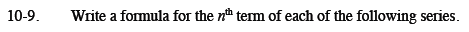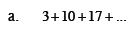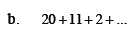Home > CCA2 > Chapter 10 > Lesson 10.1.1 > Problem10-9

10-9.
1. Write a formula for the nth term of each of the following series. Homework Help ✎

1. 3 + 10 + 17 + …

2. 20 + 11 + 2 + …The terms are increasing by 7 each time.

t(1) = 3

t(n) = 3 + 7(n − 1)

Simplified, this becomes t(n) = 7n − 4.What is added to each term to get the next?

What is the first term?